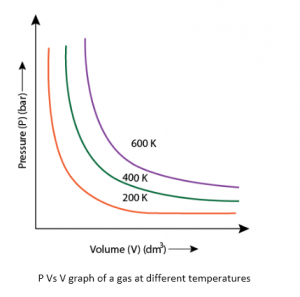# Pressure Vs Volume Relationship : Boyle's Law

In 1662, Robert Boyle experimentally found a relationship between volume and pressure (Boyle’s law). He studied the relationship between volume and pressure with the help of air and mercury at room temperature. In his experiment, he used mercury and a simple U shaped tube.

He started applying pressure, by putting more mercury in the open limb. The volume enclosed by air is the space above mercury in the shorter limb. This volume decreases when we put some more mercury. He observed that volume decreases when pressure increases. After this experiment he concluded a relationship between pressure and volume which is also known as Boyle’s law. It is one of the fundamental gas laws in chemistry.

Know more about Boyle’s Law Calculator

“At a given temperature, the volume of a fixed amount of gas is inversely proportional to the pressure provided”.

Mathematically it can be stated as:

$V ∝ \frac{1}{p}$ (For a fixed mass at constant temperature)

Or, $V$ = $k \frac{1}{p}~ or ~PV$=$k$                (k is the constant of proportionality)

• PV = Constant at fixed temperature

Where, P- Pressure

V – Volume of the gas

‘k’ is the constant which is also known as Boyle’s constant. Value of k depends on the temperature of gas, amount of gas and on the units in which pressure and volume are expressed.

Suppose P1 and V1 are the initial pressure and volume of the given mass of gas. This gas now undergoes expansion at a fixed temperature and the pressure and volume changes to P2 and V2. Now, According to Boyle’s law:

P1V1 = P2V2 (at constant temperature)

This can be explained as: “At a fixed temperature, the product of volume and pressure for a fixed amount of mass of a gas is always constant”.

Know more about Boyle’s Law Formula

Graphical verification of Boyle’s law:

Fig.1 represents the equation PV = k.

In this, each curve shows pressure and volume relationship at a constant temperature and is called as an Isotherm.P Vs V graph of a gas at different temperatures

Fig.2 is a graphical presentation between P and 1/V.

Usually the graph shows a straight line, but at high pressure gases do not follow the Boyle’s law and straight line is not obtained in the graph.